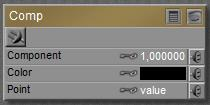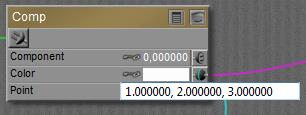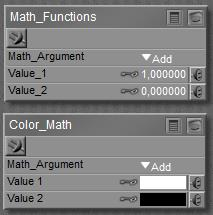# What are the Component and Math_functions / Color_math nodes used for?

Nodes are the essential building blocks in the Advanced interface to the Poser Material Room. They are the graphical representation of mathematical function calls, that is: calculation procedures which turn parameters (inputs) to a result (output).

The Math group offers various nodes that can adjust and combine colors, images and other texture elements. This article discusses:

• Component
• Math_functions
• Color_math

which split and combine value or color / image inputs in a mathematical way. See this overview of the Math group.

### ComponentThis node accepts a vector, either representing a color (Red, Green, Blue) or a pointer in space (Variable nodes, like P,N and dPdu etc.). From that vector it picks the first, second or third Component (using values 0, 1 or 2 for that) and presents that value as a result.

How do the inputs work together to make an output?

• When something is plugged into the Color slot, that takes precedence over anything else. The input is filtered (multiplied) by the color swatch, and the appropriate component of that RGB value triplet is passed through. All Point information is ignored.
• When nothing is plugged into the Color slot, but something is plugged into the Point slot instead, the appropriate component of that input is passed through. The Point value and the Color swatch are both ignored.
•When nothing is plugged into any slot, the appropriate component of the Color swatch is passed through, the Point value is ignored, unless…
• Nothing is plugged in and the Color swatch is complete black (0,0,0),then the appropriate component of the Point value is passed through. Note that this value is a value triplet (point vector) of some sort.

### Math_Functions and Color_Math

Both nodes produce a result from a math operation on two inputs. They both offer the same list of choices for such operations. The difference between them is:

• Math_Functions produces a single value, and turns every input into a single value as well before the operation is performed. So, when I offer it a color or a pointing vector, that is turned into a value first (for colors: the brightness it calculated and used). The math is performed as one might be familiar with, just using function calls or performing algebra and the like.
• Color_Math produces a three-component vector, based on such color- or pointing vectors for input as well. Single values are turned into a vector with three equal components, like a value is turned into a greyscale tone. The math is performed on a per-component basis, that’s the Math_Function operation three times:
• Result 0 = math operation on inputA comp 0 and inputB comp 0
• Result 1 = math operation on InputA comp 1 …
• Result 2 = …Most functions do what I can expect from them, as defined by any basic math textbook. See the Poser Reference Manual on them in case of doubt. Some operations need both inputs (like Add and Multiply), other operations work on Input_1 only (like sine, square root).

The Smoothstep, Bias and Gain operations expect an image for Input-1. Smoothstep then will perform the bicubic enhancement which is about standard when an image is resized and resaved in my imaging software. Bias and Gain expect a value (or three-value vector) for Input_2 as well, and will adjust image brightness (Bias) and contrast (Gain) accordingly. The value 0.5 is considered neutral (no net effect), lower values (down to 0) reduce brightness/contrast, and higher values (up to 1) increase them. Negative contrasts will invert the image input.

Note that all images and all color swatches can be affected by the Gamma mechanism (Poser 10, or all Pro versions) at render time, and hence the outcome will be affected as well, unless explicit measures are taken to prevent that.  Images should have their Gamma value set to 1.0 and swatches should have brightness 1.0 (that is: at least one color component should equal 100%).

Next >

This site uses Akismet to reduce spam. Learn how your comment data is processed.